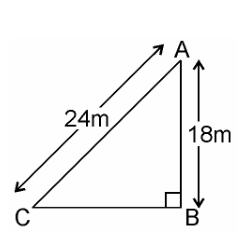# A guy wire attached to a vertical pole of height 18 m is 24 m long and has a stake attached to the other end. How far from the base of the pole should the stake be driven so that the wire will be taut?

Given

A guy wire attached to a vertical pole of height 18 m is 24 m long and has a stake attached to the other end.

Find out

The distance of the foot of the ladder from the base of the wall.

SolutionAB is pole = 18m

AC is wire = 24m

BC = ?

In right ∆ABC, by Pythagoras theorem

(AC)= (AB)+ (BC)2

(24)= (18)+ (BC)2

576 = 324 + (BC)2

576 – 324 = (BC)2

252 = (BC)2

√252 = BC

√(2 * 2 * 3 * 3 * 7) = BC

2 * 3√7 = BC

6√7 = BC

∴ Hence, the stake may be placed at distance of 6√7 from the base of the pole.(3)(0)